Finding General Solutions

Chapter 3 Class 11 Trigonometric Functions
Concept wise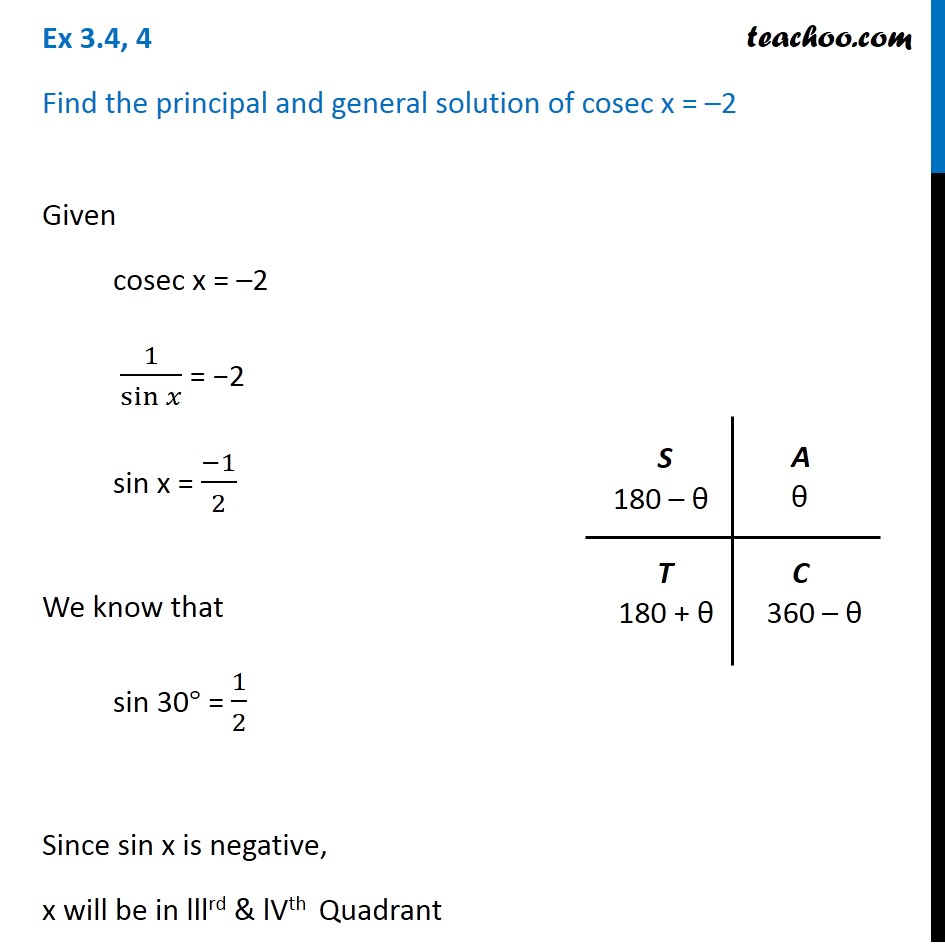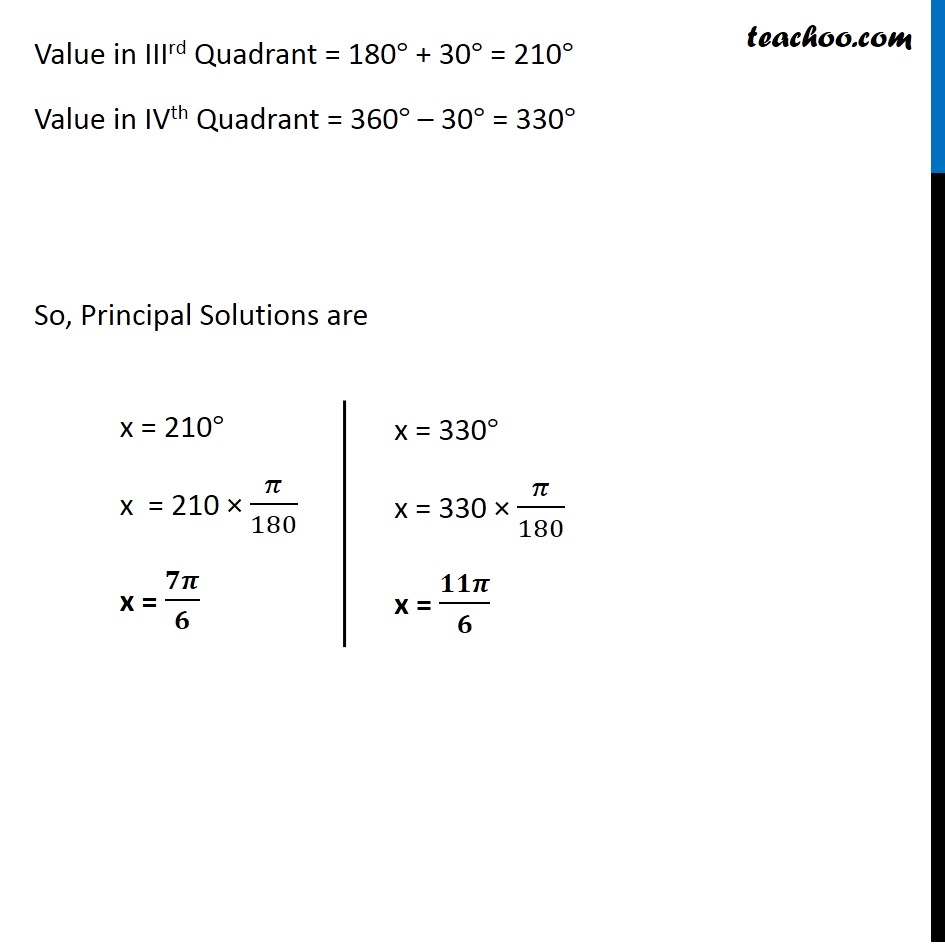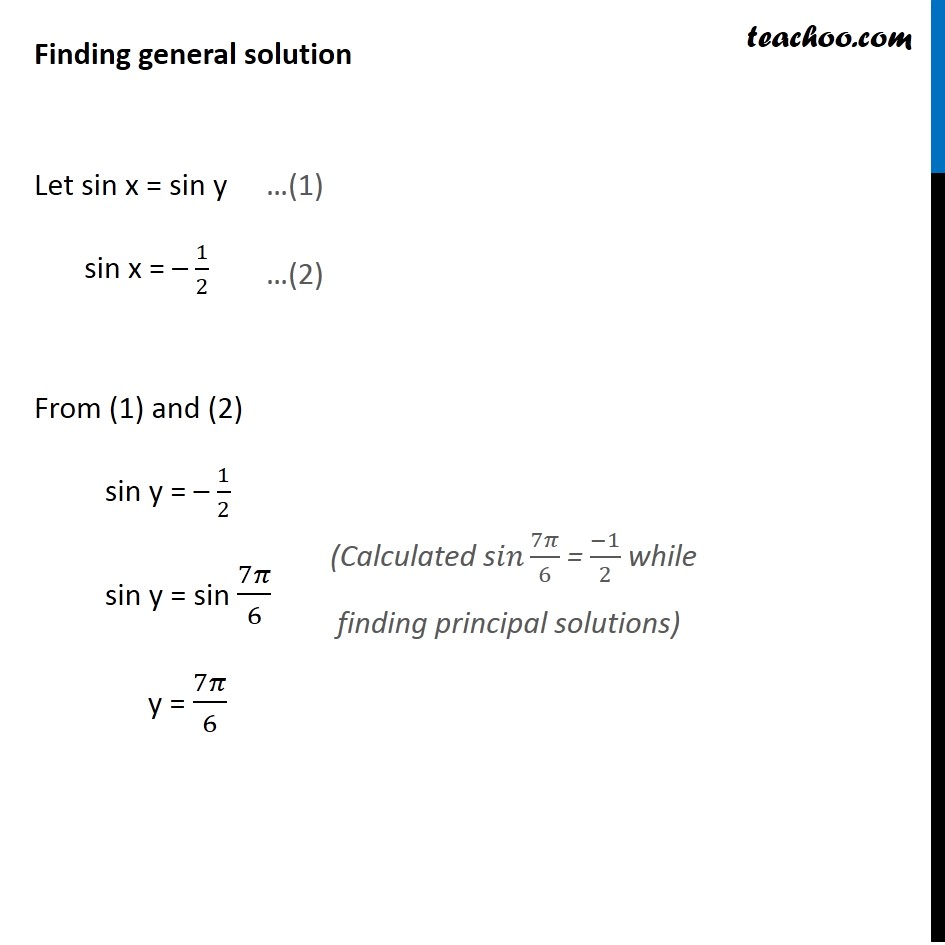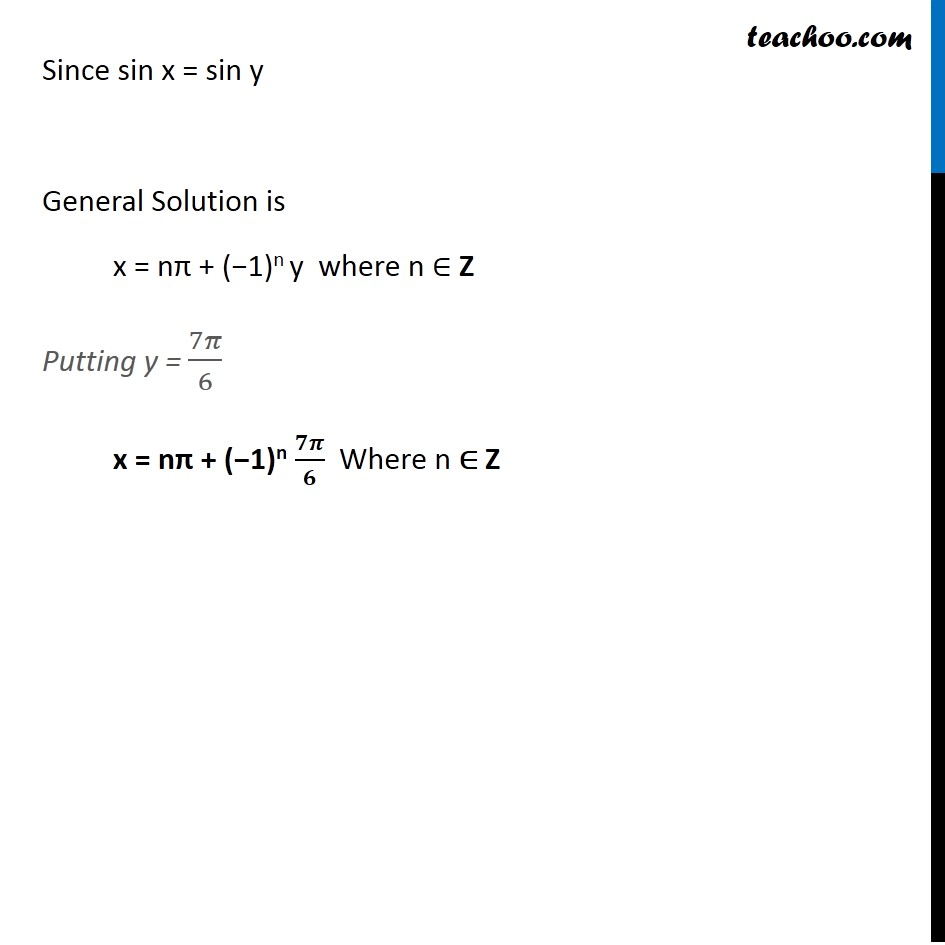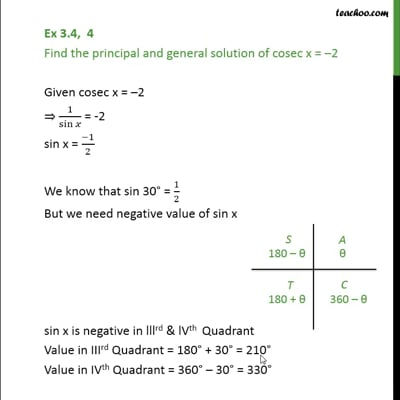This video is only available for Teachoo black users

Introducing your new favourite teacher - Teachoo Black, at only ₹83 per month

### Transcript

Ex 3.4, 4 Find the principal and general solution of cosec x = –2 Given cosec x = –2 1/sin⁡𝑥 = −2 sin x = (−1)/2 We know that sin 30° = 1/2 Since sin x is negative, x will be in lllrd & lVth Quadrant Value in IIIrd Quadrant = 180° + 30° = 210° Value in IVth Quadrant = 360° – 30° = 330° So, Principal Solutions are Finding general solution Let sin x = sin y sin x = – 1/2 From (1) and (2) sin y = – 1/2 sin y = sin 7𝜋/6 y = 7𝜋/6 (Calculated s𝑖𝑛 7𝜋/6 = (−1)/2 while finding principal solutions) Since sin x = sin y General Solution is x = nπ + (−1)n y where n ∈ Z Putting y = 7𝜋/6 x = nπ + (−1)n 𝟕𝝅/𝟔 Where n ∈ Z